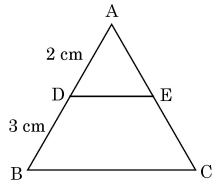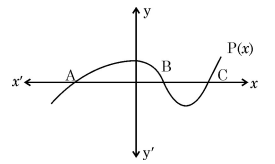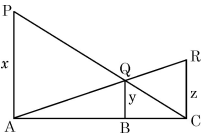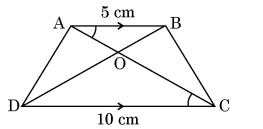GeeksforGeeks App
Open AppBrowser
Continue

# CBSE Class 10 Maths Term 1 Exam 2021 Paper Analysis Standard

The CBSE Class 10 Mathematics Term 1 Exam for session 2021-22 was conducted by the Central Board of Secondary Education (CBSE) on 4th December 2021, Saturday for both Basic and Standard Mathematics Level. This year, more than 21 lakh students appeared for CBSE Class 10 Term 1 exam 2021. The Maths exam is the most important and tough exam for CBSE Class 10th students. Therefore, considering that CBSE has divided the Question paper into two Levels: Basic and Standard.

In 2019, the CBSE introduced two-level math (Standard and Basic) in Class 10 for the first time.

• Standard Maths is for those who wants to opt Mathematics as a subject in Classes 11 and 12.
• Basic Maths is for those who do not want to pursue the subject further.

This new approach was designed to make it easier for students who do not want to continue Mathematics in higher grades.

According to the guidelines, “Students who want to continue studying Mathematics in Grades 11 and 12 must pass the Mathematics Standard paper in Grade 10. If a student then changed their mind and wanted to study Math in Class 11, they were required to take the Class 10 Standard Mathematics paper in the compartment examinations.”

The CBSE Class 10 Maths Term 1 Paper had MCQ-type questions designed in different formats like questions based on assertion-reason, case study, and competency-based questions. The paper was of 40 marks with 40 questions to be attempted out of the given 50 questions. The question paper was divided into three sections as follows:

Teachers and experts who have reviewed the CBSE Class 10 Maths Standard and Basic examinations see it as a Balanced Paper. Only approximately 10-15% of the questions were difficult yet manageable, according to them. Conceptual questions were included in both standard and basic papers. The paper may have been easily solved by students who had a solid understanding of the fundamental concepts.

Hence, the question paper was long and relatively tough for the majority of students.

Here, the Solved Questions Paper (along with the Answers and the explanation in-depth for each question) of the Standard Maths CBSE Class 10th Term 1 Exam is provided as:

### Term- I

Time allowed- 90 minutes

Maximum Marks- 40

General Instructions

1. This question paper contains 50 questions out of which 40 questions are to be attempted. All questions carry equal marks.
2. The question paper consists of three Sections – Section A, B, and C.
3. Section – A contains 20 questions. Attempt any 16 questions from Q. No. 01 to 20.
4. Section – B also contains 20 questions. Attempt any 16 questions from Q. No. 21 to 40.
5. Section – C contains two Case Studies containing 5 questions in each case. Attempt any 4 questions from Q. No. 41 to 45 and another 4 from Q. No. 46 to 50.
6. There is only one correct option for every Multiple Choice Question (MCQ). Marks will not be awarded for answering more than one option.
7. There is no negative marking.

### SECTION – A

Question Numbers 1 to 20 are of 1 mark each. Any 16 Questions from Question 1 to 20 are needed to be attempted.

Question 1: The exponent of 5 in the prime factorization of 3750 is

(a) 3

(b) 4

(c) 5

(d) 6

Question 2: The graph of a polynomial P(x) cuts the x-axis at 3 points and touches it at 2 other points. The number of zeroes of P(x) is

(a) 1

(b) 2

(c) 3

(d) 5

Question 3: The values of x and y satisfying the two equations 32x + 33y = 34, 33x + 32y = 31 respectively are :

(a) –1, 2

(b) –1, 4

(c) 1, –2

(d) –1, –4

Question 4: If A(3, 3), B(0, 0) and C(3, k) are the three vertices of an equilateral triangle ABC, then the value of k is

(a) 2

(b) –3

(c) – 3

(d) – 2

Question 5: In figure, DE || BC, AD = 2 cm and BD = 3 cm, then ar (ΔABC) : ar (ΔADE) is equal to(a) 4 : 25

(b) 2 : 3

(c) 9 : 4

(d) 25 : 4

Question 6: If cot(θ) = 1/√3, the value of sec2θ+ cosec2θ is:

(a) 1

(b) 40/9

(c) 38/9

(d) 5⅓

Question 7: The area of a quadrant of a circle where the circumference of the circle is 176 m, is

(a) 2464 m2

(b) 1232 m2

(c) 616 m2

(d) 308 m2

Question 8: For an event E, P(E) + P(–E) = x, then the value of x3 – 3 is

(a) –2

(b) 2

(c) 1

(d) –1

Question 9: What is the greatest possible speed at which a girl can walk 95 m and 171 m in an exact number of minutes?

(a) 17 m/min

(b) 19 m/min

(c) 23 m/min

(d) 13 m/min

Question 10: In the figure, the graph of a polynomial P(x) is shown. The number of zeroes of P(x) is(a) 1

(b) 2

(c) 3

(d) 4

Question 11: Two lines are given to be parallel. The equation of one of the lines is 3x – 2y = 5. The equation of the second line can be

(a) 9x + 8y = 7

(b) –12x – 8y = 7

(c) –12x + 8y = 7

(d) 12x + 8y = 7

Question 12: Three vertices of a parallelogram ABCD are A(1, 4), B(–2, 3) and C(5, 8). The ordinate of the fourth vertex D is

(a) 8

(b) 9

(c) 7

(d) 6

Question 13: In ΔABC and ΔDEF, ∠F = ∠C, ∠B = ∠E and AB = 1/2 DE. Then, the two triangles are

(a) Congruent, but not similar.

(b) Similar, but not congruent.

(c) Neither congruent nor similar.

(d) Congruent as well as similar.

Question 14: In ΔABC right angled at B, sinA = 7/25, then the value of cosC is

(a) 7/25

(b) 24/25

(c) 7/24

Question 15: The minute hand of a clock is 84 cm long. The distance covered by the tip of the minute hand from 10:10 am to 10:25 am is

(a) 44 cm

(b) 88 cm

(c) 132 cm

(d) 176 cm

Question 16: The probability that the drawn card from a pack of 52 cards is neither an ace nor a spade is

(a) 9/13

(b) 35/52

(c) 10/13

(d) 19/26

Question 17: Three alarm clocks ring their alarms at regular intervals of 20 min, 25 min, and 30 min respectively. If they first beep together at 12 noon, at what time will they beep again for the first time?

(a) 4:00 pm

(b) 4:30 pm

(c) 5:00 pm

(d) 5:30 pm

Question 18: A quadratic polynomial, the product and sum of whose zeroes are 5 and 8 respectively is

(a) k [x2 – 8x + 5]

(b) k [x2 + 8x + 5]

(c) k [x2 – 5x + 8]

(d) k [x2 + 5x + 8]

Question 19: Points A (–1, y) and B(5, 7) lie on a circle with center O (2, –3y). The values of y are

(a) 1, –7

(b) –1, 7

(c) 2, 7

(d) –2, –7

Question 20: Given that secθ = 2, the value of 1 + tanθ/sinθ is

(a) 2√2

(b) √2

(c) 3√2

(d) 2

### SECTION – B

Question numbers 21 to 40 are of 1 mark each. Any 16 Questions from Question 21 to 40 are needed to be Attempted:

Question 21: The greatest number which when divides 1251, 9377, and 15628 leaves remainder 1, 2, and 3 respectively is

(a) 575

(b) 450

(c) 750

(d) 625

Question 22: Which of the following cannot be the probability of an event?

(a) 0.01

(b) 3%

(c) 16/17

(d) 17/16

Question 23: The diameter of a car wheel is 42 cm. The number of complete revolutions it will make in moving 132 km is

(a) 104

(b) 105

(c) 106

(d) 103

Question 24: If θ is an acute angle and tanθ + cotθ = 2, then the value of sin3θ + cos3θ is

(a) 1

(b) 1/2

(c) 2√2

(d) √2

Question 25: The ratio in which the line 3x + y – 9 = 0 divides the line segment joining the points (1, 3) and (2, 7) is

(a) 3:2

(b) 2:3

(c) 3:4

(d) 4:3

Question 26: If x – 1 is a factor of the polynomial p(x) = x3 + ax2 + 2b and a + b = 4, then

(a) a = 5, b = –1

(b) a = 9, b = –5

(c) a = 7, b = –3

(d) a = 3, b = 1

Question 27: If a and b are two coprime numbers, then a3 and b3 are

(a) Coprime

(b) Not coprime

(c) Even

(d) Odd

Question 28: The area of a square that can be inscribed in a circle of area 1408/7 cm2 is

(a) 321 cm2

(b) 642 cm2

(c) 128 cm2

(d) 256 cm2

Question 29: If A (4, –2), B (7, – 2), and C (7, 9) are the vertices of a ΔABC, then ΔABC is

(a) equilateral triangle

(b) isosceles triangle

(c) right-angled triangle

(d) isosceles right-angled triangle

Question 30: If α, β are the zeros of the quadratic polynomial p(x) = x2 – (k + 6)x + 2(2k – 1), then the value of k, if α + β = 1/2 αβ, is

(a) –7

(b) 7

(c) –3

(d) 3

Question 31: If n is a natural number, then 2(5n + 6n) always ends with

(a) 1

(b) 4

(c) 3

(d) 2

Question 32: The line segment joining the points P(–3, 2) and Q(5, 7) is divided by the y-axis in the ratio

(a) 3 : 1

(b) 3 : 4

(c) 3 : 2

(d) 3 : 5

Question 33: If a cotθ + b cosecθ = p and b cotθ + a cosecθ = q, then p2 – q2

(a) a2 – b2

(b) b2 – a2

(c) a2 + b2

(d) b – a

Question 34: If the perimeter of a circle is half to that of a square, then the ratio of the area of the circle to the area of the square is

(a) 22 : 7

(b) 11 : 7

(c) 7 : 11

(d) 7 : 22

Question 35: A dice is rolled twice. The probability that 5 will not come up either time is

(a) 11/36

(b) 1/3

(c) 13/36

(d) 25/36

Question 36: The LCM of the two numbers is 2400. Which of the following CANNOT be their HCF?

(a) 300

(b) 400

(c) 500

(d) 600

Question 37: In fig., PA, QB, and RC are each perpendiculars to AC. If x = 8 cm and z = 6 cm, then y is equal to(a) 56/7 cm

(b) 7/56 cm

(c) 25/7 cm

Question 38: In a ΔABC, ∠A = x°, ∠B = (3x – 2)°, ∠C = y°. Also ∠C – ∠B = 9°. The sum of the greatest and the smallest angles of this triangle is

(a) 107°

(b) 135°

(c) 155°

(d) 145°

Question 39. If secθ + tanθ = p, then tanθ is

(a) p2 + 1/2p

(b) p2 – 1/2p

(c) p2 – 1/p2 + 1

(d) p2 + 1/p2 – 1

Question 40: The base BC of an equilateral ΔABC lies on the y-axis. The coordinates of C are (0, –3). If the origin is the mid-point of the base BC, what are the coordinates of A and B?

(a) A ( 3, 0), B(0, 3)

(b) A (±3 3, 0), B(3, 0)

(c) A (±3 3, 0), B(0, 3)

(d) A (– 3, 0), B(3, 0)

### SECTION – C

Question number 41 to 45 and 46 to 50 are based on Case Study-I and Case Study-II. Here Any 4 Questions from each Case Study are needed to be attempted

Case Study-I

A book store shopkeeper gives books on rent for reading. He has a variety of books in his store related to fiction, stories, and quizzes, etc. He takes a fixed charge for the first two days and an additional charge for the subsequent day. Amruta paid ₹22 for a book and kept it for 6 days; while Radhika paid 16 for keeping the book for 4 days.

Assume that the fixed charge be ₹x and the additional charge (per day) be ₹y.

Based on the above information, answer any four of the following questions:

Question 41: The situation of amount paid by Radhika, is algebraically represented by

(a) x – 4y = 16

(b) x + 4y = 16

(c) x – 2y = 16

(d) x + 2y = 16

Question 42: The situation of amount paid by Amruta, is algebraically represented by

(a) x – 2y = 11

(b) x – 2y = 22

(c) x + 4y = 22

(d) x – 4y = 11

Question 43: What are the fixed charges for a book?

(a) ₹ 9

(b) ₹10

(c) ₹ 13

(d) ₹15

Question 44: What are the additional charges for each subsequent day for a book?

(a) ₹ 6

(b) ₹ 5

(c) ₹ 4

(d) ₹ 3

Question 45: What is the total amount paid by both, if both of them have kept the book for 2 more days?

(a) ₹ 35

(b) ₹ 52

(c) ₹ 50

(d) ₹ 58

Case Study – II

A farmer has a field in the shape of a trapezium, whose map with a scale of 1 cm = 20 m, is given below :

The field is divided into four parts by joining the opposite vertices.Based on the above information, answer any four of the following questions :

Question 46. The two triangular regions AOB and COD are

(a) Similar by AA criterion

(b) Similar by SAS criterion

(c) Similar by RHS criterion

(d) Not similar

Question 47: The ratio of the area of the ΔAOB to the area of ΔCOD, is

(a) 4 : 1

(b) 1 : 4

(c) 1 : 2

(d) 2 : 1

Question 48: If the ratio of the perimeter of ΔAOB to the perimeter of ΔCOD would have been 1 : 4, then

(a) AB = 2 CD

(b) AB = 4 CD

(c) CD = 2 AB

(d) CD = 4 AB

Question 49: If in Δs AOD and BOC, AO/BC = AD/BO = OD/OC, then

(a) ΔAOD ∼ ΔBOC

(b) ΔAOD ∼ ΔBCO

(d) ΔODA ∼ ΔOBC

Question 50: If the ratio of areas of two similar triangles AOB and COD is 1 : 4, then which of the following statements is true?

(a) The ratio of their perimeters is 3 : 4.

(b) The corresponding altitudes have a ratio 1 : 2.

(c) The medians have a ratio 1 : 4.

(d) The angle bisectors have a ratio 1 : 16.